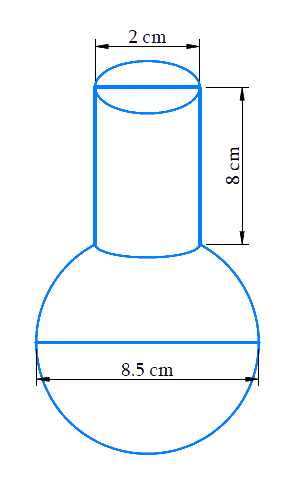# Ex.13.2 Q8 Surface Areas and Volumes Solution - NCERT Maths Class 10

Go back to  'Ex.13.2'

## Question

A spherical glass vessel has a cylindrical neck $$8\rm\,{ cm}$$ long, $$2\rm\,{ cm}$$ in diameter; the diameter of the spherical part is $$8.5\rm\,{ cm}$$. By measuring the amount of water it holds, a child finds its volume to be $$345\rm\,{ cm^3}$$. Check whether she is correct, taking the above as the inside measurements, and $$\pi = 3.14.$$

Video Solution
Surface Areas And Volumes
Ex 13.2 | Question 8

## Text Solution

What is known?

A spherical glass vessel with a cylindrical neck of length $$=8\rm\,{ cm}$$ and diameter $$=2\rm\,{ cm}$$

The diameter of the spherical part $$=8.5\rm\,{ cm}$$

According to the child volume of the vessel $$345\rm{cm}^3$$

What is unknown?

Whether the child is correct in saying the volume of the glass vessel is $$345\,{\text{c}}{{\text{m}}^3}$$

Reasoning:

Draw a figure to visualize the glass vesselSince the glass vessel is a combination of a sphere and a cylinder

Volume of glass vessel $$=$$ Volume of the spherical part $$+$$ Volume of the cylindrical part

Steps:

Height of cylindrical part,$$h = 8 \rm cm$$

Radius of cylindrical part,\begin{align}{r_1} = \frac{{2 \rm cm}}{2} = 1 \rm cm\end{align}

Radius of spherical part,\begin{align}{r_2} = \frac{{8.5 \rm cm}}{2} = 4.25 \rm cm\end{align}

Volume of the glass vessel $$=$$ volume of the spherical part $$+$$ volume of the cylindrical part

\begin{align}&= \frac{4}{3}\pi r_2^3 + \pi r_1^2h\\&= \pi \left( {\frac{4}{3}r_2^3 + r_1^2h} \right)\\ &= 3.14 \!\! \times \!\!\begin{pmatrix} \frac{4}{3} \times 4.25 \rm cm \\ \times 4.25 \rm cm \\ \times 4.25 \rm cm \\ + 1 \rm cm \\ \times 1 \rm cm \\ \times 8 \rm cm \end{pmatrix} \\&= \begin{Bmatrix} 3.14 \\\times \begin{pmatrix} 102.354 \rm c{m^3} \\ + 8 \rm c{m^3} \end{pmatrix} \end{Bmatrix} \\&= 3.14 \times 110.354 \rm c{m^3}\\&= 346.51 \rm c{m^3}\end{align}

Therefore, the child is not correct in saying that the volume of the glass vessel is $$345\rm{cm}^3$$

Learn from the best math teachers and top your exams

• Live one on one classroom and doubt clearing
• Practice worksheets in and after class for conceptual clarity
• Personalized curriculum to keep up with school